# PostgreSQL Tutorial: Compare Two Tables

September 14, 2023

Summary: in this tutorial, you will learn various ways to compare two tables in PostgreSQL.

There are several ways to compare the content of two tables to find the differences between them. We will show you two commonly used techniques to compare data of two tables.

## Compare two tables using EXCEPT and UNION operators

First, let’s create two tables named `foo` and `bar`, and insert some sample data for the demonstration.

``````CREATE TABLE foo (
ID INT PRIMARY KEY,
NAME VARCHAR (50)
);
``````
``````INSERT INTO foo (ID, NAME)
VALUES
(1, 'a'),
(2, 'b');
``````
``````CREATE TABLE bar (
ID INT PRIMARY KEY,
NAME VARCHAR (50)
);
``````
``````INSERT INTO bar (ID, NAME)
VALUES
(1, 'a'),
(2, 'b');
``````

The `foo` table has the same structure and data as the `bar` table.

Next, we update one row in the `bar` table.

``````UPDATE bar
SET name = 'c'
WHERE
id = 2;
``````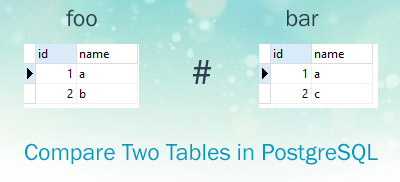Then, to find the rows in the `foo` table but not in the `bar` table, we use the following query:

``````SELECT
ID,
NAME,
'not in bar' AS note
FROM
foo
EXCEPT
SELECT
ID,
NAME,
'not in bar' AS note
FROM
bar
``````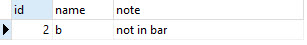We used `EXCEPT` operator that returns the rows in the `foo` table but not in the `bar` table. We can apply the same technique to find the rows that are in the `bar` table but not in the `foo` table.

``````SELECT
ID,
NAME,
'not in foo' AS note
FROM
bar
EXCEPT
SELECT
ID,
NAME,
'not in foo' AS note
FROM
foo
``````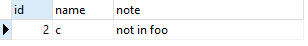Finally, we use UNION operator to combine the result of both queries to find:

• Rows in the `bar` table but not in the `foo` table.
• Rows in the `foo` table but not in the `bar` table.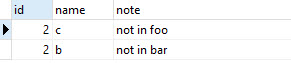## Compare two tables using OUTER JOIN

We can use the outer join to compare two tables as follows:

``````SELECT
id,
name
FROM
foo
FULL OUTER JOIN bar USING (id, name)
WHERE
foo.id IS NULL
OR bar.id IS NULL;
``````

It returns the differences between two tables:To find the number of rows that are in the `foo` table but not `bar` table and vice versa, we use the COUNT function as follows:

``````SELECT
COUNT (*)
FROM
foo
FULL OUTER JOIN bar USING (id, name)
WHERE
foo.id IS NULL
OR bar.id IS NULL;
``````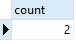In this tutorial, we have shown you two ways to compare two tables in PostgreSQL.